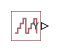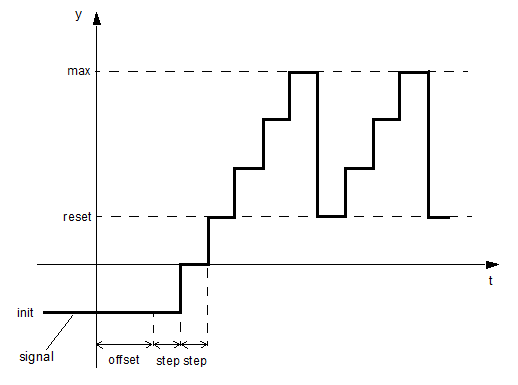# PS Counter

Increment output signal by 1 with every time step

•Libraries:
Simscape / Foundation Library / Physical Signals / Sources

## Description

The PS Counter block increments the output signal, Y, by 1 with every time step repeatedly between the minimum (reset) value and the maximum value. You can optionally specify an initial signal value, different from the reset value, and an initial time offset. The output signal generated by the block is shown in the following diagram.If the initial time offset is specified, the block outputs the initial signal value `init` until the simulation time reaches the `offset` value, at which point the counting cycle starts. The block outputs the current value for one time step, then repeatedly increments the signal value by 1 and outputs it for one time step, until it reaches the maximum value `max`. The block outputs the `max` value for one time step, then returns to the `reset` value, and the counting cycle starts again.

Use this block, in conjunction with other physical signal blocks, to model discrete behaviors.

The Discrete-Time PWM Voltage Source example illustrates how you can use the PS Counter block to build components with more complex behaviors. For an alternative asynchronous implementation, see the Asynchronous PWM Voltage Source example. The discrete-time version is better suited to fixed-step solvers and hardware-in-the-loop applications, whereas the asynchronous implementation is better suited to fast desktop simulation using variable-step solvers.

## Ports

### Output

expand all

Output physical signal.

## Parameters

expand all

The value and unit of the time `step` interval. The default `step` value is `1` s. To specify an initial time offset, enter the parameter value as ```[ step , offset ]```, otherwise the `offset` value is assumed to be `0`.

The value of the output signal at the beginning of the first counting cycle. If you specify an initial time offset by using the Sample time parameter, the output of the block remains at this value until the simulation time reaches the `offset` value, after which the first counting cycle starts. The value must be an integer.

The value of the output signal at the beginning of each counting cycle except the first one. The output of the block remains at this value for one time `step`, specified by the Sample time parameter. The value must be an integer.

The value of the output signal at the end of the counting cycle. The output of the block remains at this value for one time `step`, specified by the Sample time parameter, at which point the signal returns to the Reset value and the cycle starts again. The value must be an integer. The default value is `intmax` (2147483647, the largest positive value that can be represented in the MATLAB® software with a 32-bit integer).

## Version History

Introduced in R2012b

expand all